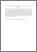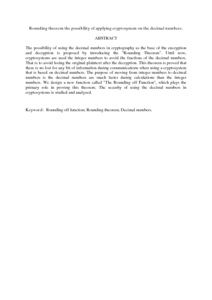# Rounding theorem the possibility of applying cryptosystem on the decimal numbers.

## Citation

Alfaris, Rand and Kamel Ariffin, Muhamad Rezal and Md Said, Mohamed Rushdan (2008) Rounding theorem the possibility of applying cryptosystem on the decimal numbers. Journal of Mathematics and Statistics, 4 (1). pp. 15-20. ISSN 1549-3644

## Abstract

The possibility of using the decimal numbers in cryptography as the base of the encryption and decryption is proposed by introducing the "Rounding Theorem". Until now, cryptosystems are used the integer numbers to avoid the fractions of the decimal numbers. That is to avoid losing the original plaintext after the decryption. This theorem is proved that there is no lost for any bit of information during communications when using a cryptosystem that is based on decimal numbers. The purpose of moving from integer numbers to decimal numbers is the decimal numbers are much faster during calculations than the integer numbers. We design a new function called "The Rounding off Function", which plays the primary role in proving this theorem. The security of using the decimal numbers in cryptosystems is studied and analyzed.Preview
PDF (Abstract)
Rounding theorem the possibility of applying cryptosystem on the decimal numbers.pdfView Item